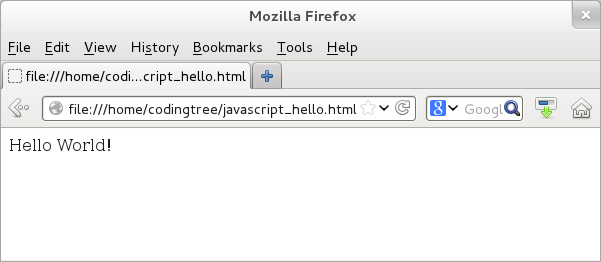## CodingBison

jQuery is built on top of JavaScript. Knowing some of the JavaScript basics would go a long way! So, let us get started with some of the basic JavaScript concepts like how to embed JavaScript code, variables, and objects.

JavaScript is a universally used client-side programming to provide client-side interaction for Internet browsers. In this role, JavaScript enables browsers to handle user interaction and to process their input.

Like jQuery, running a JavaScript program is fairly easy. We can embed JavaScript code within HTML tags "<script type="text/javascript">" and "</script>". All pieces of code that sit between these two tags are executed in the JavaScript context. There is no constraint as to where we can keep the JavaScript code on an HTML page and as to how many times we can keep the JavaScript code.

With that, let us see a trivial JavaScript example that prints "Hello World!". We start with simple HTML page and then JavaScript code within the page (let us name this file, "javascript_hello.html"). This programs prints this text on the HTML page by appending the text to an already existing "div" element of the page. It does so by using the innerHTML property of the "idDiv" HTML element. Here is the example:

``` <!doctype html>
<html>

<body>
<div id="idDiv"> </div>

<script type="text/javascript">
<!-- Needed for old-browsers that do not understand Javascript.

// Get a handle of the div element.
var elem = document.getElementById("idDiv");
elem.innerHTML +=  "Hello World! <br>";

// -->
</script>
</body>

</html>
```

Next, let us fire up the browser and point its location to the above "javascript_hello.html" file. Once the page is loaded, we would see the following output: is the output:Figure: jQuery "Hello World!" program

With JavaScript, we also have the option of including a file containing the javascript code directly using the "src" attribute of script tag. This allows us to separate HTML from JavaScript logic. The benefit of this separation-of-concerns approach is that each files (HTML and JavaScript) can evolve independently of each other and without being cluttered with content from the other type.

For example, let us create a file (let us say hello.js) and move the two JavaScript lines within the script tags.

``` // Get a handle of the div element.
var elem = document.getElementById("idDiv");
elem.innerHTML +=  "Hello World! <br>";
```

Next, we can directly source this file using the script tags. We are assuming that the "hello.js" file is in the same directory as that of the html file. Thus, the following example would also have the same "Hello World!" output.

``` <!doctype html>
<html>
<body>
<div id="idDiv"> </div>

<script type="text/javascript" src="hello.js"></script>

</body>
</html>
```

In fact, this is how we include the location of jQuery library in a file.

``` <script type="text/javascript" src="https://ajax.googleapis.com/ajax/libs/jquery/1.9.1/jquery.min.js">
</script>
```

### JavaScript Variables/Objects

We need variables to store values. Accordingly, JavaScript provides two types of variable: primitive and object types. The primitive ones are the simpler ones like numbers, strings, boolean, null, NaN (Not a Number), and undefined. The object types can hold many values; structurally, they are a collection of property/value pairs.

JavaScript numbers store both integers and floats (fractional numbers). JavaScript uses a 64 bit storage space for such number variables. The string type is simply a finite ordered sequence of 16-bit characters, where characters can be letters, numbers (from 0 to 9), white-space etc. The boolean type essentially contains two values: true and false. The null type means a lack of value for a variable. The undefined type is similar and means that even though a variable is defined, it has not been assigned any value. The NaN type represents all those values that are not numbers. One unique thing about NaN is that it is the only type that does not equal itself; thus (NaN != NaN) evaluates to true!

Unlike primitive types, object types are containers and hold property/value pairs. In fact, in JavaScript, anything that is not a primitive type, is an object. Thus, functions, arrays, regular expressions, Date etc are all objects. When adding property/value pairs to an object, we need to specify each property and value by using a colon (":") as a separator. For example, if were to define an object, objX, holding a property, propY with a value of 237, then we can do that as: "var objX = {propY: 237};".

With that, let us see an example that uses both variables and objects. It defines variables of types number and string along with an object. Next, it uses the JavaScript's typeof operator to retrieve the storage type of the variable/object. Here it is:

``` <!doctype html>
<html>
<div id="idDiv"> </div>

<script type="text/javascript">
var varNum1 = 10;               // varNum1 is a number.
var varNum2 = 10.50;            // varNum2 is a number.
var varString = "Nobel Prize";  // varString is a string.

// Get a handle of the div element.
var elem = document.getElementById("idDiv");

// Use typeof operator to print variable types.
elem.innerHTML += "varNum1 is "   + varNum1   + " (type: "   + (typeof varNum1)   + ")<br>";
elem.innerHTML += "varNum2 is "   + varNum2   + " (type: "   + (typeof varNum2)   + ")<br>";
elem.innerHTML += "varString is " + varString + " (type: "   + (typeof varString)   + ")<br>";

// Define an object.
var objNobel = {name: "Alfred Nobel", invention: "Dynamite"};

// Access properties of objNobel.
elem.innerHTML += "<br>The type of objNobel is " + (typeof objNobel)   + "<br>";
elem.innerHTML += "Value of property name: " + objNobel.name + "<br>";
elem.innerHTML += "Value of property invention: " + objNobel.invention + "<br>";
</script>

</html>
```

When we load this page on a browser, then we would see the following text output in the browser.

``` varNum1 is 10 (type: number)
varNum2 is 10.5 (type: number)
varString is Nobel Prize (type: undefined)

The type of objNobel is object
Value of property name: Alfred Nobel
Value of property invention: Dynamite
```